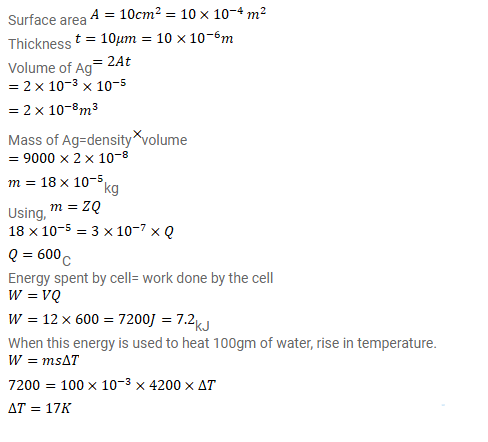# A plate of area 10 cm squer is to be electroplated with copper

Question:

A plate of area $10 \mathrm{~cm}^{2}$ is to be electroplated with copper (density $9000 \mathrm{~kg} / \mathrm{m}^{3}$ ) to a thickness of 10 micrometers on both sides, using a cell of 12V. Calculate the energy spent by the cell in the process of deposition. If this energy is used to heat $100 \mathrm{~g}$ of water, calculate the rise in the temperature of the water. ECE of copper= $3 \times 10^{-7} \mathrm{~kg} / \mathrm{C}$ and specific heat capacity of water $=4200 \mathrm{~J} / \mathrm{kg}-\mathrm{K}$.

Solution: